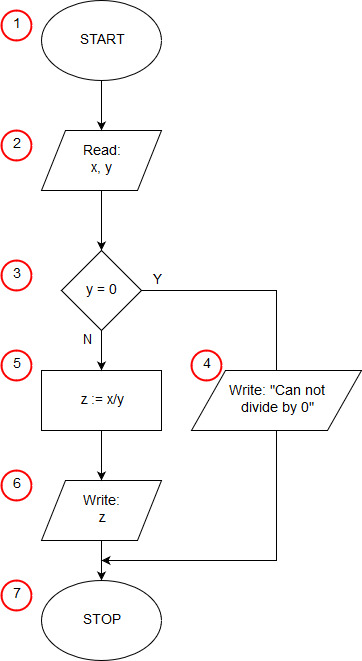# Algorithm of division in Python

<<algorithmx = float(input('x = ')) y = float(input('y = ')) if y == 0: print('Can not divide by 0') else: z = x / y print('z =',z)

In the 1st line of the program, using the input function, we read a floating-point number (of the float type) from the standard input (keyboard), which we immediately store in the x variable. The input function simultaneously displays on the screen the text given as its argument. In the 2nd line, we ask the user to enter the second number that we assign to the variable y.

The conditional statement on line 3 is the selection block (3) of our algorithm. The statement checks if y is equal to 0. When the condition is met, the print() statement indented on line 4 (input/output block (4)) is executed. When the condition is not met, the instructions indented after the else keyword from line 5 are executed, in our case these are the instructions in lines 6 and 7. First, in line 6, we assign to the variable z the value of the quotient x by y (operational block (5 )), and then in line 7 we write the result (input-output block (6)). This is where our program ends. There is no separate statement in Python that tells you when a program ends.